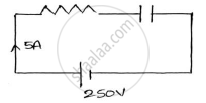# A Resistance and a Capacitor Connected in Series Across a 250v Supply Draws 5a at 50hz. When Frequency is Increased to 60hz, It Draws 5.8a. Find the Values of R and C. Also Find Active Powe - Basic Electrical and Electronics Engineering

Sum

A resistance and a capacitor connected in series across a 250V supply draws 5A at 50Hz. When frequency is increased to 60Hz, it draws 5.8A. Find the values of R & C. Also find active power and power factor in both cases.

#### Solution

𝐼1=5𝐴  V = 250V   𝑓1=50𝐻𝑧   𝑓2=60𝐻𝑧   𝐼2=5.8𝐴
(1) Values of R and C.

For 𝑓1=50𝐻𝑧   𝑍1=V/I_1=250/5= 50Ω

Z_1^2=(R^2+(1/(2pif_1c))^2)=(R^2+(1/(100piC))^2)

R^2+(1/(100piC))^2=2500…………….(1)For 𝑓1=60𝐻𝑧   Z_2=V/I_2=250/5.8 = 43.1Ω

Z_2^2=(R^2+1/(2pif_1c))=(R^2+(1/(100piC))^2)

R^2+(1/(120piC))^2=1857.9Ω …………….(2)

From (1) and (2) we get,

R = 19.96Ω C = 69.4𝝁𝑭

(2) Power draw in the both cases.
𝑃1= 𝐼12𝑅=52×19.96 = 499w    𝑃2= 𝐼22𝑅=5.82×19.96 = 671.45w
𝑷𝟏=𝟒𝟗𝟗𝒘 and 𝑷𝟐= 671.45w

(3) Power factor in both cases:-
P1=VI1cosφ1
499=250×5×cos𝜑1 => cos𝜑1=0.3992
𝝋𝟏=𝟔𝟔.𝟒𝟕𝟏°
P2=VI2cosφ2
671.45=250×5.8×cos𝜑2 => cos𝜑2=0.463
𝝋𝟏=𝟔𝟐.𝟒𝟏𝟒𝟔°

Concept: AC Through Resistance
Is there an error in this question or solution?